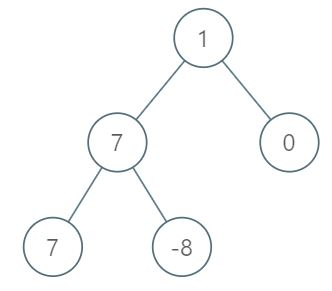All contents and pictures on this website come from the Internet and are updated regularly every week. They are for personal study and research only, and should not be used for commercial purposes. Thank you for your cooperation.

# 1161. Maximum Level Sum of a Binary Tree

Given the `root` of a binary tree, the level of its root is `1`, the level of its children is `2`, and so on.

Return the smallest level `X` such that the sum of all the values of nodes at level `X` is maximal.

Example 1:```Input: [1,7,0,7,-8,null,null]
Output: 2
Explanation:
Level 1 sum = 1.
Level 2 sum = 7 + 0 = 7.
Level 3 sum = 7 + -8 = -1.
So we return the level with the maximum sum which is level 2.
```

Note:

1. The number of nodes in the given tree is between `1` and `10^4`.
2. `-10^5 <= node.val <= 10^5`

Medium

Normal